# Character Puzzles

Character Puzzles is a problem that test imagination, cleverness and skill of the solver. Puzzles are often contrived as a form of Entertainment, But they can also be considered as mathematical or logical problems in most of cases.

Character Puzzles such as finding missing number in a series.

In this type of questions, a figure or a matrix is given in which some numbers are filled according to a rule. A place is left blank. You have to find out a character (a number or a letter) from the given possible answers which may be filled in the blank space.

LearnFrenzy provides you lots of fully solved Questions and Answers with explanation.

Some examples are given below.

Example 1:

Which number will replace the question mark ?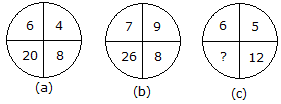Solution :

From fig. a: 6 + 4 + 8 = 18

18 + 2 = 20

From fig. b: 7 + 9 + 8 = 24

24 + 2 = 26

From fig. c: 6 + 5 + 12 = 23

23 + 2 = 25

Hence the number 25 will replace the question mark.

Example 2:

Which number will replace the question mark ?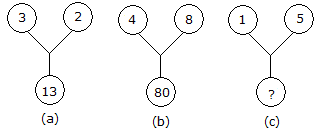Solution :

From fig. a: (3)2 + (2)2 = 13

From fig. b: (4)2 + (8)2 = 80

From fig. c: ? = (1)2 + (5)2

? = 1 + 25

? = 26

Hence the number 26 will replace the question mark.

Example 3:

Which number will replace the question mark ?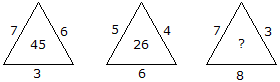Solution :

From fig. a: 7 x 6 + 3 = 45

From fig. b: 5 x 4 + 6 = 26

From fig. c: 7 x 3 + 8 = 29

Hence the number 29 will replace the question mark.

Example 4:

Which number will replace the question mark ?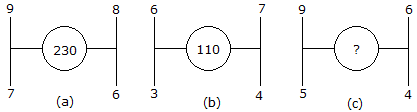Solution :

From fig. a: 92 + 82 + 7+ 62 = 81 + 64 + 49 + 36 = 230

From fig. b: 62 + 72 + 32 + 42 = 36 + 49 + 9 + 16 = 110

From fig. c: 92 + 62 + 52 + 42 = 81 + 36 + 25 + 16 = 158

Hence the number 158 will replace the question mark.

Example 5:

Which number will replace the question mark ?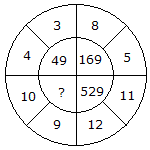Solution :

(4 + 3)2 = (7)2 = 49

(8 + 5)2 = (13)2 = 169

(11 + 12)2 = (23)2 = 529

(10 + 9)2 = (19)2 = 361

Hence the number 361 will replace the question mark.

Example 6:

Which number will replace the question mark ?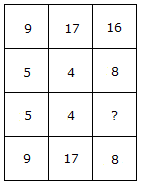Solution :

From column I: (9 x 5) % 5 = 9

From column II: (17 x 4) % 4 = 17

From column III: (16 x ?) % 8 = 8

16? = 64

? = 4

Hence the number 4 will replace the question mark.

Example 7:

Which number will replace the question mark ?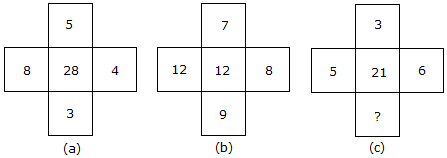Solution :

From fig. a: (8 x 5) – (4 x 3) = 28

From fig. b: (12 x 7) – (8 x 9) = 12

From fig. c: (5 x 3) – (6 x ?) = 21

15 – 6? = 21

6? = -6

? = -1

Hence the number -1 will replace the question mark.

Example 8:

Which number will replace the question mark ?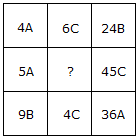Solution :

In each row there are ‘A’, ‘B’ and ‘C’

In second row ‘A’ and ‘C’ are already there

Hence in place of ?, there will be ‘B’.

From first row : 4A x 6C = 24B

From third row : 9B x 4C = 36A

From second row : 5A x ? = 45C

? = (45C/5A)

? = 9B

Hence the number 9B will replace the question mark.

Example 9:

Which number will replace the question mark ?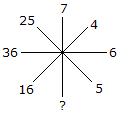Solution :

(5)= 25

(6)2 = 36

(4)2 = 16

(7)2 = 49

Hence the number 49 will replace the question mark.